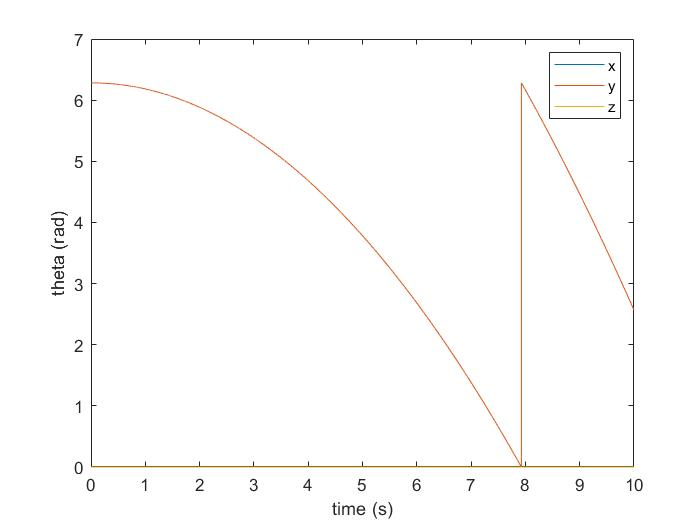30 Jan

#### Implementation of Rotation

The following steps are used to update the dynamics of an aerial robot.

1. Calculate the rotation matrix R from the last iteration's Euler Angle θ.
2. Calculate angular acceleration given current force and torque inputs, and the angular velocity from last iteraion.
3. Update angular velocity
4. Calculate Euler angle rate with old Euler angle and new angular velocity
5. Update Euler angle
6. Calculate acceleration and integrate to update position

I wrote a new script to test the implementation. The Euler angle with 0.001 N of constant thrust from propeller is shown here: